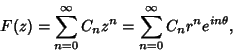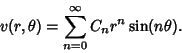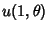## Abel's Theorem

Given a Taylor Series(1)

where the Complex Numberhas been written in the polar form, examine the Real and Imaginary Parts(2)(3)

Abel's theorem states that, ifandare Convergent, then(4)

Stated in words, Abel's theorem guarantees that, if a Real Power Series Converges for some Positive value of the argument, the Domain of Uniform Convergence extends at least up to and including this point. Furthermore, the continuity of the sum function extends at least up to and including this point.

References

Arfken, G. Mathematical Methods for Physicists, 3rd ed. Orlando, FL: Academic Press, p. 773, 1985.